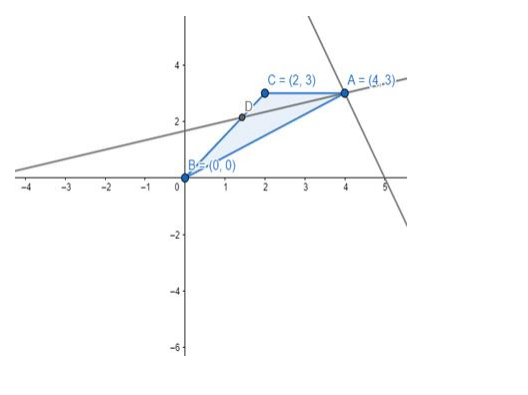# If A(4, 3), B(0, 0) and C(2, 3) are the vertices of a`
Question:

If A(4, 3), B(0, 0) and C(2, 3) are the vertices of a ΔABC, find the equation of the bisector of ∠A.

Solution:

Construction: Draw a line from vertex A intersecting side BC of the triangle at D (as there is one bisector for exterior angle also but it is the default that we have to find interior angle bisector).As the angle between the sides AB and angle bisector AD and side AC and angle bisector AD is equal.

$\angle \mathrm{A}=2 \theta \Rightarrow \angle \mathrm{BAD}=\angle \mathrm{CAD}=\theta$

Then using the angle between two lines, if the slope of AD be m and slope of AB

Slope of $A B=\frac{y_{2}-y_{1}}{x_{2}-x_{1}} \Rightarrow \frac{3-0}{4-0}=\frac{3}{4}$

Putting the values in the equation

$\tan \theta=\frac{\mathrm{m}_{2}-\mathrm{m}_{1}}{1+\mathrm{m}_{1} \cdot \mathrm{m}_{2}}$ .................................(1)

$\Rightarrow \frac{\frac{3}{4}-\mathrm{m}}{1+\mathrm{m} \cdot \frac{3}{4}}=\frac{\frac{3-4 \mathrm{~m}}{4}}{\frac{4+3 \mathrm{~m}}{4}}$

$\tan \theta=\frac{3-4 \mathrm{~m}}{4+3 \mathrm{~m}}$    ..........................(2)

Again for side AC slope

Slope of $A C=\frac{y_{2}-y_{1}}{x_{2}-x_{1}} \Rightarrow \frac{3-3}{2-4}=0$

Putting in equation (1)

$\tan \theta=\frac{\mathrm{m}_{2}-\mathrm{m}_{1}}{1+\mathrm{m}_{1} \cdot \mathrm{m}_{2}} \Rightarrow \frac{\mathrm{m}-0}{1+0 \cdot \mathrm{m}}=\mathrm{m}$ ..……………….(3)

From equation (2) and (3),we have

$\mathrm{m}=\frac{3-4 \mathrm{~m}}{4+3 \mathrm{~m}} \Rightarrow 4 \mathrm{~m}+3 \mathrm{~m}^{2}+4 \mathrm{~m}-3=0$

$3 m^{2}+8 m-3=0$

From equation we have two values of $\mathrm{m}-3, \frac{1}{3}$

tanθ = - 3 as tanx is negative in II and IV quadrant means it is obtuse angle either way(exterior here)we require interior angle so will consider the positive value of m.

$\mathrm{m}=\tan \theta=\frac{1}{3}$

As we obtained the slope of angle bisector which passes through A vertex so using slope intercept form first calculate the value of the intercept

$y=m x+c$ .................................(4)

$3=\frac{1}{3}(4)+c \Rightarrow c=3-\frac{4}{3} \Rightarrow c=\frac{9-4}{3} \Rightarrow \frac{5}{3}$

Putting the value of c in equation (4),we have

$y=\frac{1}{3} x+\frac{5}{3} \Rightarrow x-3 y+5=0$

So, the required equation of angle bisector is x - 3y + 5 = 0.